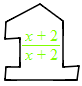### Home > CCA2 > Chapter 3 > Lesson 3.2.4 > Problem3-103

3-103.

Use the methods developed in class to add or subtract the following rational expressions. Be sure to look for factors before trying to determine a common denominator, and simplify your answers, if possible.

1. $\frac { 4 x } { x ^ { 2 } - 2 x - 8 } + \frac { 4 } { x - 4 }$

This is addition of fractions. What are the steps for adding fractions?

Factor.

$\frac{4x}{(x+2)(x-4)} +\frac{4}{x-4}$

To make common denominators, multiply the second term by the Giant One below.$\frac{4x}{(x+2)(x-4)}+\frac{4(x+2)}{(x+2)(x-4)}$

Add the numerators and keep the denominators the same.

$\frac{8x+8}{(x+2)(x-4)}$

2. $\frac { 16 x - 12 } { 4 x ^ { 2 } + 5 x - 6 } - \frac { 3 } { x + 2 }$

Refer to part (a).

$\frac{1}{x+2}$